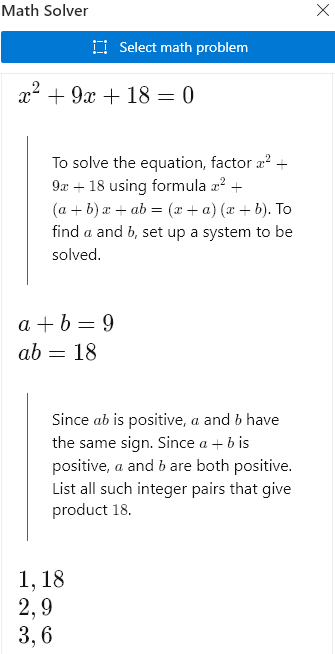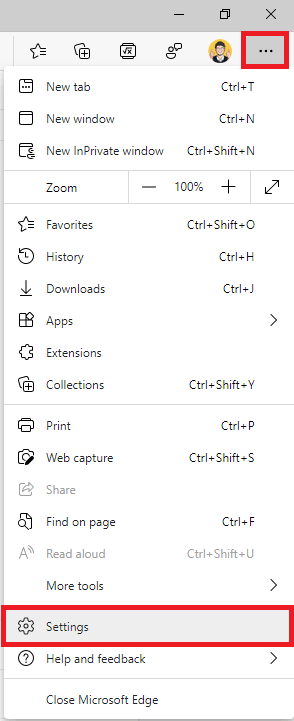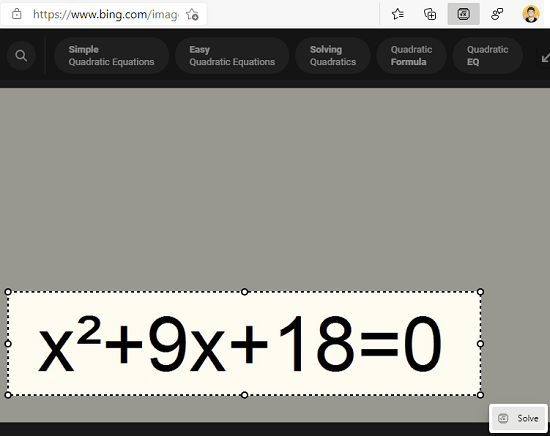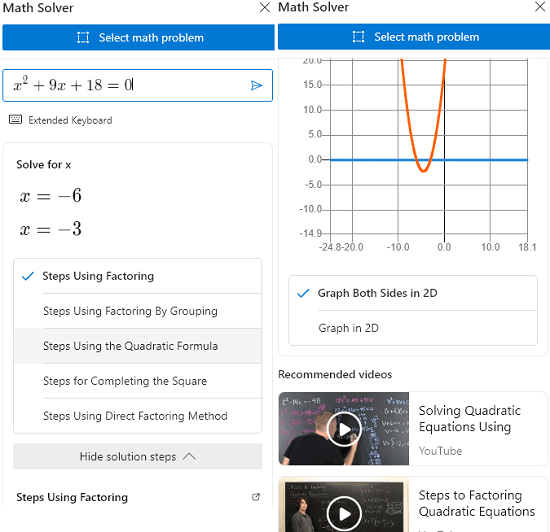Editor Ratings:
User Ratings:
[Total: 0 Average: 0]

Math Solver is a new toolbar added to Microsoft Edge that lets you solve mathematical equations you come across while browsing the web. It lets you select an equation using a rectangular snipping tool and then solves it online. It shows you step-by-step solution for the equation with some helpful resources. Here it opens a sidebar in the current window where it displays the solution of the equation and there is no sign up required for it. All you have to do it to enable it first and then you can start using it right from the Edge toolbar.

Math solver is a great tool for high school or engineering students. It uses the online version of Math Solver to solve equations that you specify in it. There is even an Android app for Math Solver tool and now you can use it in the Edge browser as well. It works flawlessly and the OCR technique that it uses to identify the equation is remarkable. With minimal intervention, it can produce accurate results and can help you learn/solve math.## How to Enable Math Solver in Microsoft Edge and Use it?

For now, you can try your hands on this new Math Solver tool of Edge in the Canary build. This feature is not available by default to all users. So, if your Canary build has it then you can enable it as follows.

• Step 1: Open Edge Settings by clicking on the 3 dots icon at top right corner.• Step 2: Go to “Appearance” section. Turn on the “Show Math Solver button” option.• Step 3: Now, you will have the Math Solver icon in the Edge toolbar and it looks like this.Now, you have successfully enabled and Math Solver and it is now time to use it. To do that, you only have to go to a webpage that has some math equation. After that, you just click the icon of Math Solver and then select the equation.Next, it will process the captured equation and will solve it for you. You can see the step-by-step solution in the sidebar. Not only that but it even consolidates related resources and videos to the equation you selected in the same sidebar.This is it, In this way, you can use this simple and very useful tool of Microsoft Edge. I am not exactly sure when will it arrive in the stable release but I bet this will be a very useful feature for schools and university students.

### Wrap up…

As a math lover I really liked this Math Solver tool of Edge. It is great and I wish that I had it when I was in high school so that I could’ve saved many hours that I spent scratching my head while doing quadratic equations. So, if you are a math lover like me then give it a try and let me know what you think.

 Editor Ratings: User Ratings:[Total: 0 Average: 0] Tags: math equation